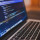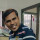Brainy Puzzles
Puzzle Solvers: Interesting brainy puzzles shared by fellow puzzlers
407 Members
Join this group to post and comment.# 5,8,13,?,34,55,89 Find the missing numberMissing number is 21

5,8,13,?,34,55,89

5+8=13

34+55=89

so, 8+13=X

X = 21

In the given sequence,in order to get a number we need to add previous two numbers.

>> 5,8,13,21,34,55,89PIYUSH KUSHWAHA • Sep 5, 2019

@Aswini cse .. good job... Or you can subtract the previous two number to get your answer... Like 89-55=34, 55-34=21, 34-21=13, 21-13=8, 13-8=5.... :PMissing number is 21